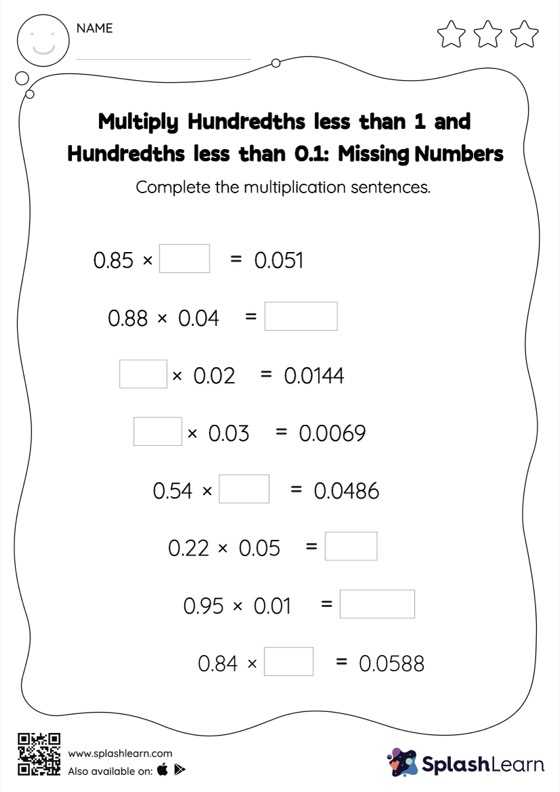# Multiply Hundredths less than 1 and Hundredths less than 0.1: Missing Numbers Worksheet

Home > Multiply Hundredths less than 1 and Hundredths less than 0.1: Missing NumbersChildren practice multiplying hundredths less than 1 and hundredths less than 0.1 With this worksheet. Students first multiply as though there is no decimal when multiplying two decimals. They then add the same number of digits after the decimal point in the product as there are decimal digits in each number. Multiply hundredths less than 1 and hundredths less than 0.1 Worksheet encourages them to apply this understanding to find the missing number in decimal multiplication.